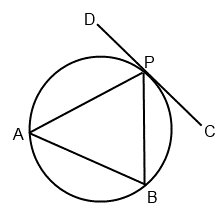+91-22-62437100 / 01 | help@reachivy.com

# GMAT Problem Solving

GMAT problem solving section may seem simple as they use 8th grade math concepts. But often students are stumped by the complexity of the questions. Also, the challenge of managing time adds its own difficulties. Here is a quick problem solving test that simulates the GMAT. Take the test now!

To begin click Next

Question 1:

If the volume of a right circular cylinder is reduced by 60% by reducing the height of the cylinder by 10%, by what percent must the radius of the base be reduced?

Question 2:

How many ways can the eleven letters in the word MISSISSIPPI be arranged to create unique 11-letter codes if all the letters are used in each code?

Question 3:

If f(a) = a2, what is the value of (f(a + b) – f(a))/b?

Question 4:

A rectangular solid has dimensions of 3 x 5 x 7. What is the length of the longest segment whose endpoints are vertices of the solid?

Question 5:

Eight senators and six representatives have volunteered to work on a Congressional committee that will consist of two members from each body. How many unique committees can be created?

Question 6:

The volume of a cone is 1/3πr2h and the volume of a sphere is 4/3πr3, where r = radius and h = height. If a right circular cone whose radius is 4 is inscribed in a sphere with a radius of 5, what fraction of the sphere’s volume is the cone’s volume, approximately?

Question 7:

The circumference of a right circular cylinder is half its height. The radius of the cylinder is x. What is the volume of the cylinder in terms of x?

Question 8:If AP = BP, and arc AP = 140, what is the measure of angle BPC?

Question 9:

If ABC is an equilateral triangle, B is equidistant from all points on the sector outside triangle ABC, and AC = 12, what is the area of the shaded region?

Question 10:

In an aquarium tank, the ratio of the number of clownfish to zebrafish is 7 to 56. If 6 more zebrafish were added to the tank, the new ratio would be 7 to 58. How many total clownfish and zebrafish will be in the tank after the zebrafish are added?

You have answered all the questions in the quiz. Press Submit to view your score